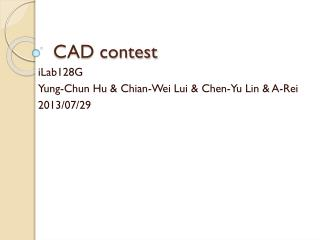Download PresentationDownload Presentation- - - - - - - - - - - - - - - - - - - - - - - - - - - E N D - - - - - - - - - - - - - - - - - - - - - - - - - - -
##### Presentation Transcript

1. CAD contest iLab128G Yung-Chun Hu & Chian-Wei Lui & Chen-Yu Lin & A-Rei 2013/07/29

2. Outline • Introduction • Main idea • Adder • Flow & progress • Experimental results • Future work

3. Main idea - Adder • Divide macro blocks into standard cells PI PI …. n-bit Adder 1-bit Adder CO CI …. Sum Sum

4. Main idea - Adder A Sum B Find XOR in circuit directly

5. Main idea - Adder The rest part of Adder A sum 1 A ~A

6. Main idea - Adder adder n-bit adder inverter PI 00 00 A1 00 … X X ~A X Sum

7. Libraries • assign out = &in; • assign out = |in; • assign out = ^in; • assign out = ~&in; • assign out = ~|in; • assign out = ~^in; • assign out = in2[in1]; • assign out = in1 + in2; • assign out = in1 + in2 + in3; • assign out = in1 + in2 + in3 + in4; • assign out = in1 * in2; • assign out = (in1 + in2) * in3; • assign out = in1 * in2 + in3 * in4; • assign out = in1 * in2 + in3 * in4 + in5 * in6; Simplifiedto adder/multiplier

8. Flow & Progress Parser (including design & library) Library type Hybrid Adder/Index/Multiplier Boolean Logic Step1: Adder/Multiplier Step1: Standard Cells Step1: Finding And Step2: Mapping Step2: Mapping Step2: Mapping Optimization (Resyn2 & ??) Output & Verification

9. Experimental results

10. Future work • Improve continuous AND structure • Mapping adder • Finding a good way to map multiplier